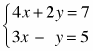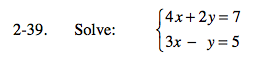### Home > PC > Chapter 2 > Lesson 2.2.1 > Problem2-39

2-39.

Solve:Homework Help ✎1. Double the second equation and add it to the first.

4x + 2y = 76
x − 2y = 10
10x = 17

2. Solve for x.
3. Then substitute that answer back into one of the original equations.
4. Check your answer by substituting both answers for x and y into the other original equation.